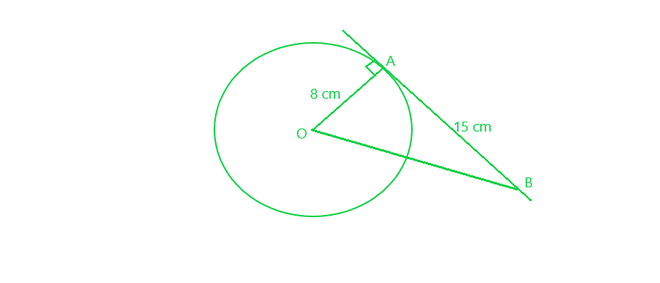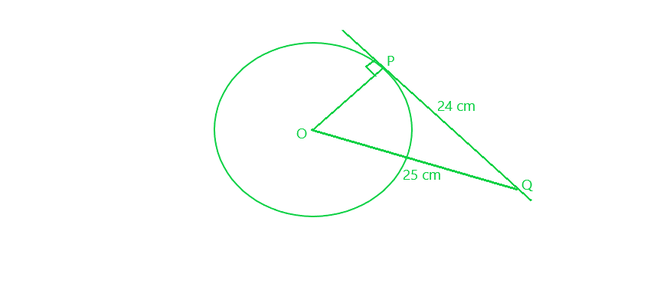GeeksforGeeks App
Open AppBrowser
Continue

## Related Articles

• RD Sharma Class 10 Solutions

# Class 10 RD Sharma Solutions – Chapter 10 Circles – Exercise 10.1

### (i) The common point of a tangent and the circle is called ……….

Solution:

Point of contact.

Solution:

Two

Solution:

One

Solution:

Secant

### (v) The angle between tangent at a point on a circle and the radius through the point is ………..

Solution:

Right angle (90°)

### Question 2. How many tangents can a circle have?

Solution:

Tangent is a line that intersect a circle at only point. Since there are a infinite number of points on a circle, a circle can have infinite tangents.

### Question 3. O is the centre of a circle of radius 8 cm. The tangent at a point A on the circle cuts a line through O at B such that AB = 15 cm. Find OB.

Solution:AB = 15 cm

OA ⊥ tangent AB

Therefore, In right ∆OAB, by applying Pythagoras Theorem:

OB² = OA² + AB²

=> OB² = (8)² + (15)²

= 64 + 225 = 289 = (17)²

=> OB = 17 cm

Thus, OB = 17 cm

### Question 4. If the tangent at a point P to a circle with centre O cuts a line through O at Q such that PQ = 24 cm and OQ = 25 cm. Find the radius of the circle.

Solution:OQ = 25 cm

PQ = 24 cm

OP ⊥ tangent PQ

therefore, In right ∆OPQ, by applying Pythagoras Theorem:

OQ² = OP² + PQ²

=> (25)² = OP² + (24)²

=> 625 = OP² + 576

=> OP² = 625 – 576 = 49

=> OP² = (7)²

OP = 7 cm

Thus, radius of the circle is 7 cm

My Personal Notes arrow_drop_up
Related Tutorials